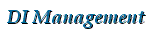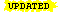DI Management Home > Mathematics > Transforming a matrix to reduced row echelon form

#Transforming a matrix to reduced row echelon form

This page shows how to transform a matrix to reduced row echelon form (RREF) also called row canonical form.[Updated 2023-06-26]

Please try our matrix calculator which does this transformation and shows the workings: see Transform matrix to row canonical form.

First, some theory.

## Row equivalent matrices and elementary row operations

Affiliate disclosure: we get a small commission for purchases made through the above links

A matrix A is said to be row equivalent to a matrix $B$, written $A \sim B$, if $B$ can be obtained from $A$ by a sequence of elementary row operations.

Suppose $A$ is a $n \times m$ matrix with rows $r_1, r_2, \ldots, r_n$,

$A = \begin{bmatrix} \mathbf{r_1} \\ \mathbf{r_2} \\ \vdots \\ \mathbf{r_n} \end{bmatrix} = \begin{bmatrix} a_{11} & a_{12} & \cdots & a_{1m}\\ a_{21} & a_{22} & \cdots & a_{2m}\\ \vdots & \vdots & \ddots & \vdots \\ a_{n1} & a_{n2} & \cdots & a_{nm} \end{bmatrix},$

then the following operations on $A$ are called elementary row operations.

$[R_1]$ (Row Interchange)
Interchange rows $r_i$ and $r_j$. We write $r_i \leftrightarrow r_j$.
$[R_2]$ (Row Scaling)
Replace row $r_i$ by a nonzero multiple of itself. We write $r_i \to kr_i$.
$[R_3]$ (Row Addition)
Replace row $r_j$ by the sum of itself and a nonzero multiple of row $r_i$. We write $r_j \to r_j + kr_i$.

You can read the arrow $\to$ as "is replaced by". Other authors may show this in a different way.

## Row canonical form/Reduced row echelon form/RREF

Echelon
(military) a formation in which units follow one another but are offset sufficiently to allow each unit a clear line of fire ahead.
A step-like formation of troops.
Any structure or group of structures arranged in a steplike form.

In other words, in echelon formation we arrange things so the row behind you won't shoot you.

Definition A matrix $A$ is said to be in row canonical form or reduced row echelon form (RREF) if the following conditions hold:

1. All zero rows, if any, are at the bottom of the matrix.
2. Each leading nonzero entry in a row is to the right of the leading nonzero entry in the preceding row.
3. Each pivot (leading nonzero entry) is equal to 1.
4. Each pivot is the only nonzero entry in its column.

where a leading nonzero element of a row of $A$ is the first nonzero element in the row [LIPS08].

An example of a reduced row echelon matrix isThe pivots are shown circled. Note that every element to the left of a pivot is zero, and every other element in the column with the pivot is zero. Put another way, if we imagine the matrix as an army marching up the page, then a soldier at any pivot can fire his gun forwards (or left or backwards) and he won't hit one of his own side.

## Why is this important?

We can deduce many useful facts about a matrix once it has been transformed to its canonical form. Let $A$ denote a general matrix and let $E$ denote its RREF form.

Every matrix $A$ is row equivalent to a unique matrix $E$ in row canonical (RREF) form.
This means the form and elements of $E$ are uniquely determined by $A$. So, if two matrices $M$ and $N$ both reduce to the same row canonical form $E$, then $M\sim N$.
The rank of a matrix $A$ is equal to the number of pivots in $E$.
This is one definition of rank. We write this as $\text{rank}(A)$ or $\text{rk}(A)$. The rank of a matrix is equal to the dimension of both the row space and the column space.
All invertible matrices reduce to the identity matrix $I$.
If the $n \times n$ matrix $A$ is invertible, then $E$ is always the $n \times n$ identity matrix.

## Computing the inverse of a matrix and solving the system Ax = b

We can also use the row reduction process to compute the inverse $A^{-1}$ of a matrix and to compute the solution of the system $A\mathbf{x}=\mathbf{b}$.

To do this we use the fact that, if $A$ is an $n \times n$ invertible matrix and $I$ is the $n \times n$ identity matrix, then $[A \mid I] \xrightarrow{RREF} [I \mid A^{-1}]$ If $\mathbf{b}$ is an $n \times 1$ vector and we want to solve the system $A\mathbf{x} = \mathbf{b}$, then $[A \mid \mathbf{b}] \xrightarrow{RREF} [I \mid \mathbf{x}]$

The notation $[P \mid Q]$ denotes an augmented matrix (Augment, v., to make greater, add more of the same thing). This is simply the two matrices written side-by-side as a larger matrix (they must have the same number of rows). The vertical line is just to help us visualise the two parts. We can ignore it if we want to.

For example, if $A = \begin{bmatrix} 1 & 2 & 1 \\ 2 & 3 & -1 \\ 3 & -2 & -1 \end{bmatrix}$ then $[A \mid I]= \left[ \begin{array}{ccc|ccc} 1 & 2 & 1 & 1 & 0 & 0 \\ 2 & 3 & -1 & 0 & 1 & 0 \\ 3 & -2 & -1 & 0 & 0 & 1 \end{array} \right] \xrightarrow{RREF} \left[ \begin{array}{ccc|ccc} 1 & 0 & 0 & 1/4 & 0 & 1/4 \\ 0 & 1 & 0 & 1/28 & 1/7 & -3/28 \\ 0 & 0 & 1 & 19/28 & -2/7 & -1/28 \end{array} \right] = [I \mid A^{-1}],$ which gives us the inverse of $A$, $A^{-1} = \begin{bmatrix} 1/4 & 0 & 1/4 \\ 1/28 & 1/7 & -3/28 \\ 19/28 & -2/7 & -1/28 \end{bmatrix}.$ And if $\mathbf{b} = \begin{bmatrix} 3 \\ -4 \\ 5 \end{bmatrix}$ then $[A \mid \mathbf{b}]= \left[ \begin{array}{ccc|c} 1 & 2 & 1 & 3 \\ 2 & 3 & -1 & -4 \\ 3 & -2 & -1 & 5 \end{array} \right] \xrightarrow{RREF} \left[ \begin{array}{ccc|c} 1 & 0 & 0 & 2 \\ 0 & 1 & 0 & -1 \\ 0 & 0 & 1 & 3 \end{array} \right] = [I \mid \mathbf{x}],$ which gives us the solution of $A \mathbf{x} = \mathbf{b}$, $\mathbf{x} = \begin{bmatrix} 2 \\ -1 \\3 \end{bmatrix}.$

This only works if $A$ is invertible. So, if you do not get the identity matrix on the left, then either $A^{-1}$ does not exist or there is not a unique solution to the linear system $A\mathbf{x}=\mathbf{b}$.

## The algorithm

We describe here the Gauss-Jordan elimination procedure, which is simpler to implement and carries out the complete reduction in one pass. There is a more efficient method, usually called Gaussian elimination, which produces the same result in two stages (forward elimination and backward substition). For more details of the latter see Algorithm 3.1 in [LIPS08].

A quick description of the Gauss-Jordan method in words is:

1. Deal with each row $i$ from $1$ to $n$ in turn, and keep a counter $j$ of columns starting at 1 and progressing rightwards to $m$.
2. Find the next column j with a nonzero entry in $A(i,j)$ or below, skipping any column with zero entries from row $i$ to $n$.
3. Interchange rows, if necessary, so that the pivot element $A(i,j)$ is nonzero. [Row interchange]
4. Make the pivot equal to 1 by dividing each element in the pivot row $i$ by the value of the pivot. [Row scaling]
5. Make all elements above and below the pivot equal to 0 by subtracting a suitable multiple of the pivot row from each other row. [Row addition]
6. Repeat for all rows.

Algorithm: Transforming a matrix to row canonical/reduced row echelon form (RREF).
INPUT: $n \times m$ matrix $A$.
OUTPUT: $n \times m$ matrix in reduced row echelon form.
1. Set $j \leftarrow 1$.
2. For each row $i$ from 1 to $n$ do
1. While column $j$ has zero elements in rows $i$ to $n$, set $j \leftarrow j+1$. If $j > m$ return $A$.
2. If element $a_{ij}$ is zero, then interchange row $i$ with a row $x > i$ that has $a_{xj} \neq 0$.
3. Divide each element of row $i$ by $a_{ij}$, thus making the pivot $a_{ij}$ equal to one.
4. For each row $k$ from 1 to $n$, with $k \neq i$, subtract row $i$ multiplied by $a_{kj}$ from row $k$.
5. Set $j \leftarrow j+1$. If $j > m$ break.
3. Return transformed matrix $A$.

There are many, many variants of the above algorithm. For example, in step 2a you could always select the largest element of the column as the pivot to help reduce rounding errors, and you could combine steps 2c and 2d.

## Matrix calculator

Did we mention that we have our own matrix calculator which does this transformation and shows the workings? See Transform matrix to row canonical form.

## Coding theory matrix calculator

See also our other matrix calculator Transform coding theory generator matrix to to standard form. This carries out similar matrix operations to transform a generator matrix or parity-check matrix to standard form over a Galois field. The terms generator matrix and parity-check matrix are concepts used in coding theory. Students of coding theory could use this matrix calculator to check their assignment answers. Lazy students could even let it do the work for them, but we obviously disapprove of that.